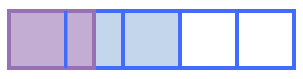### Home > MC1 > Chapter 6 > Lesson 6.2.3 > Problem6-71

6-71.
1. . Calculate each of the following parts of parts. Homework Help ✎

1.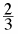of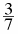2.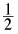of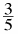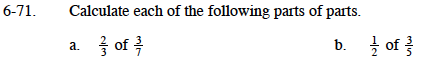Draw a diagram to represent three-sevenths.

Since the green shaded region is three pieces, you can see that two-thirds of this region would be the blue shaded region.

$\frac{2}{7}$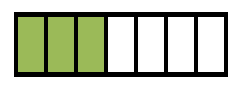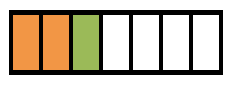Below is a diagram representing one-half of three-fifths.